iTHOR API Reference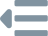Installation
Concepts
Initialization
Scenes
Object Types
Set Object States
Domain Randomization
Object Movement
Object State ChangesThe figure above shows AI2-THOR's agent-simulator interaction loop. Our backend scenes, actions, and observations are stored within Unity, a powerful real-time game engine. From the Python side, we sequentially interact with Unity by taking actions using AI2-THOR's Python

Controller
.

The returned data after executing any action is an

Event
. Each
Event
contains a ton of information to encode the state of the environment after the action has been executed:event = controller.step(...)
References the last executed Eventcontroller.last_event
Response
<ai2thor.server.Event

.frame: {...}
.cv2img: {...}

.depth_frame: {...}

.instance_segmentation_frame: {...}
.instance_detections2D: {...}

.color_to_object_id: {...}
.object_id_to_color: {...}
/>


### Event Response

RGB frame of the scene from the agent egocentric perspective. The size of the frame is the initialized (

height
,
width
, 3) stored in the
numpy.uint8
format.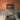Same as the

frame
, except image channels are in BGR ordering. This is often useful with Python's OpenCV module (i.e.,
cv2
), which expects images with BGR ordering.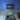Measures the distance, in meters, from the agent to each pixel in the frame. The size of the frame is the initialized (

height
,
width
) stored in the
numpy.float32
format.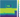Remark

Upon

initialization
or
reset
,
renderDepthImage
must be set to
True
in order for the depth frame to appear.

Segments every object instance in the scene. The size of the frame is (

height
,
width
, 3) stored in the
numpy.uint8
format. Each unique pixel color corresponds to a different object, which are indexable with
color_to_object_id
or
object_id_to_color
.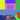Remark

Upon

initialization
or
reset
,
renderInstanceSegmentation
must be set to
True
in order for the instance segmentation frame to appear.

Segments every object instance in the scene, storing each segmentation as a boolean image mask.

The keys are

objectId
s visible in the frame and each value is the boolean
numpy.ndarray
of size (
height
,
width
) that segments the image.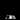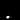Remark

Upon

initialization
or
reset
,
renderInstanceSegmentation
must be set to
True
in order for the instance masks to appear.

Provides the 2D bounding box around every object instance in the scene.

The keys are object IDs and the values are [Upper Left $x$, Upper Left $y$, Lower Right $x$, Lower Right $y$], where each element is the number of pixels it is from the top left corner of the image.

Remark

Upon

initialization
or
reset
,
renderInstanceSegmentation
must be set to
True
in order for the 2D instance detections to appear.

Provides a mapping from each pixel's color, represented as a hashable tuple, in the

instance_segmentation_frame
to what object it represents.

Same as

color_to_object_id
, except for the keys and values being swapped.

The metadata dictionary contains summary information about the state of the environment.event.metadata
Response
{
"errorMessage": {...}
"lastActionSuccess": {...}
"actionReturn": {...}
"lastAction": {...}

"sceneName": {...}
"sceneBounds": {...}

"agent": {...}
"objects": {...}

"fov": {...}
"screenWidth": {...}
"screenHeight": {...}
{...}
}


If an action fails, feedback is provided as to what caused the failure.

States whether the last action was able to successfully execute.

An exception will not be raised upon invalid states. For instance, if an agent is standing right in-front of a wall and tries to

, it will fail, but an exception will not be raised.

If an action is unsuccessful, the state of the environment will not have changed.

Holds the resulting data for actions that query the environment, such as

GetReachablePositions
.

The name of the action passed into the

Controller
.

The name of the scene that the agent is currently located in.

This object provides all coordinates that are within bounds of the scene. This can be used in tandem with actions like

PlaceObjectAtPoint
to make sure the coordinate used is not out of bounds. This returns a
sceneBounds
object that includes an 8x3 matrix of xyz coordinates that represent the 8 corners of the box encompassing the entire scene, an xyz dictionary for the coordinates of the center of that box, and an xyz dictionary for the size (extents) of that box. Example:{
"center": {
'x': -1.45,
'y': 1.407,
'z': 0.2
},
"cornerPoints": [
[ 1.5,   2.92,  3.2],
[ 1.5,   2.92, -2.8],
[ 1.5, -0.106,  3.2],
[ 1.5, -0.106, -2.8],
[-4.4,   2.92,  3.2],
[-4.4,   2.92, -2.8],
[-4.4, -0.106,  3.2],
[-4.4, -0.106, -2.8]
],
"size": {
"x": 5.9,
"y": 3.02,
"z": 6.0
}
}

Information about the pose of the agent. See Agent Metadata for more.

agent
key contains the pose of the agent after the action has executed.event.metadata["agent"]
Response
{
cameraHorizon: {...},
isStanding: {...},
position: {...},
rotation: {...},
{...}
}


The angle in degrees that the camera's pitch is rotated.

Warning

Negative camera

horizon
values correspond to the agent looking up, whereas positive
horizon
values correspond to the agent looking down.

True
if the agent is currently in a standing position, otherwise
False
. This bool can be changed if the agent uses the
Stand
or
Crouch
actions.

Warning

The

default
agent is currently the only agent with the ability to stand.

The global position of the agent, with keys for $x$, $y$, and $z$.

Remark
The $y$ coordinate corresponds to upwards in 3D space.

The local rotation of the agent's body, with keys for $x$ (pitch), $y$ (yaw), and $z$ (roll). Since the

default
agent's body can only change its yaw rotation, both $x$ and $z$ will always be approximately $0$.

Each object has a plethora of information exposed about it in each event.

Remark
Beyond what is shown here, the object metadata also provides information for each object state change action, which is documented on the Object State Changes page.event.metadata["objects"][i]
Response
{
"objectId": {...},
"objectType": {...},
"name": {...},

"distance": {...},
"visible": {...},

"position": {...},
"rotation": {...},

"axisAlignedBoundingBox": {...},
"objectOrientedBoundingBox": {...},

"mass": {...},
"salientMaterials": {...},

"parentReceptacles": {...},
"receptacle": {...},
"receptacleObjectIds": {...},

"ObjectTemperature": {...},
"canChangeTempToHot": {...},
"canChangeTempToCold": {...},

"moveable": {...},
"isMoving": {...},

"pickupable": {...},
"isPickedUp": {...},

{...Object State Changes...}
}


The unique ID of each object in the scene. It is generated at runtime and composed of an object's

objectType
and
position
.

Example:

AlarmClock|-02.08|+00.94|-03.62
.

The annotated type of the object. Each time is specified in the

Object Types
section.

Name of the object in Unity Scene. These names are unique within any individual scene.

The Euclidean distance from near the center-point of the object to the agent's camera.

Indicates whether the object is visible, and within the initialized visibility distance of the agent.

Warning

The visible property does not mean the object is literally visible in the frame. Rather, the object both has to be visible and at a distance of less than the initialized

visibilityDistance
away.

The global position of the object, with keys for $x$, $y$, and $z$.

Note that $y$ corresponds to the upward coordinate in 3D space.

The local rotation of the object, with keys for $x$ (pitch), $y$ (yaw), and $z$ (roll).

Returns an axisAlignedBoundingBox object that includes an 8x3 matrix of xyz coordinates that represent the 8 corners of the box, an xyz dictionary for the coordinates of the center of the box, and an xyz dictionary for the size (extents) of the box. This axis aligned bounding box is the smallest box that can completely encloses the sim object that is aligned to the world axis. This means that if the object is rotated or moved, the overall size and volume of this axis aligned box will change in order to remain aligned relative to the static, world-axis. This is best used for rough approximations of the area/volume an object takes up. Do note that large, oddly shaped objects like CounterTops that wrap around kitchens may have awkwardly defined axis-aligned bounds. Example:{
"center": {
"x": -1.336,
"y":  1.098,
"z":  0.221
},
"cornerPoints": [
[-1.232, 1.277, 0.319],
[-1.232, 1.277, 0.124],
[-1.232, 0.919, 0.319],
[-1.232, 0.919, 0.124],
[ -1.44, 1.277, 0.319],
[ -1.44, 1.277, 0.124],
[ -1.44, 0.919, 0.319],
[ -1.44, 0.919, 0.124],
],
"size": {
"x": 0.208,
"y": 0.358,
"z": 0.195
}
}

Returns an objectOrientedBoundingBox object that includes an 8x3 matrix of xyz coordinates that represent the 8 corners of the object oriented box. This object oriented bounding box is a box that completely encloses a sim object. The difference between this object oriented box and the axis aligned box above is this box’s dimensions are static relative to the object’s rotation and position. This means this object oriented box will always have the same total volume regardless of how the object is manipulated/rotated/moved. Note that only Pickupable objects have an objectOrientedBoundingBox at this time. Example:{
'cornerPoints': [
[-1.445, 0.910, 0.115],
[-1.228, 0.910, 0.115],
[-1.228, 0.910, 0.328],
[-1.445, 0.910, 0.328],
[-1.445, 1.284, 0.115],
[-1.228, 1.284, 0.115],
[-1.228, 1.284, 0.328],
[-1.445, 1.284, 0.328]
]
}

The mass of a Pickupable or Moveable sim object in Kilograms

Array of strings listing the salient materials a pickupable object is composed of. Valid strings are: Metal, Wood, Plastic, Glass, Ceramic, Stone, Fabric, Rubber, Food, Paper, Wax, Soap, Sponge, and Organic.

A list of objectId strings of all receptacles that contain this object.

If True, this object has the Receptacle property and can contain other objects.

If the object is a receptacle, this is an array of objectIds that the receptacle contains.

String that indicates what this object’s current abstracted temperature is. Valid strings are: Hot, Cold, RoomTemp.

If True, this object is a source of Heat and can contextually change other object’s Temperature to Hot.

If True, this object is a source of Cold and can contextually change other object’s Temperature to Cold.

If True, this object has the Moveable property and can be moved around the environment with actions like PushObject.

A bool tracking if this object is actively in motion. This can be useful when tracking object behaviors while using PausePhysicsAutoSim and AdvancePhysicsStep actions.

If True, this object has the Pickupable property and can be picked up by the agent with the PickupObject action.

Only used if this object is pickupable = True. If True, this object is currently being picked up by the agent. If False, the object is not being held by the agent.

Environment queries are actions that query the environment to extract additional metadata regarding the current state. Since there is a performance cost that comes from calculating each environment query, they are not automatically provided in the metadata after each action.

Query actions do not alter the state of the environment. Thus, they are often substantially faster than non-query actions (e.g., MoveAhead, RotateRight), since image frames and object metadata can be reused from the previous Event.

GetObjectInFrame
queries the current view of the agent for the object that appears at specified $(x, y)$ coordinate, relative to its current view. If there is an object that appears at the coordinate, its objectId is provided in
. Alternatively, if no object is at the provided coordinate,
will be
None
and
bool(query)
will be
False
.

This action can be used in tandem with object interaction actions, where

GetObjectInFrame
is first called to extract an
objectId
, and if the objectId is extracted.query = controller.step(
action="GetObjectInFrame",
x=0.64,
y=0.40,
checkVisible=False
)

object_id = query.metadata["actionReturn"]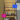### Get Coordinate from Raycast Parameters

#### x

: float
required

The $x$ coordinate from the current image frame, corresponding to the relative distance from the left of the frame. Valid values are in $[0:1]$.

The $y$ coordinate from the current image frame, corresponding to the relative distance from the top of the frame. Valid values are in $[0:1]$.

If

False
, the returned object will only be provided if it is within a distance of the initialized
visibilityDistance
(default: 1.5 meters) from the agent. This is set to
False
by default so that the agent can only interact with objects in-front of it, rather than objects far across the room.

GetCoordinateFromRaycast
sends a raycast out from the camera in the direction of the $(x, y)$ screen coordinate, relative to the agent's current view. The world $(x, y, z)$ coordinate of the first point of collision that is hit on an object by the raycast is returned in
.query = controller.step(
action="GetCoordinateFromRaycast",
x=0.64,
y=0.40
)

coordinate = query.metadata["actionReturn"]### Get Coordinate from Raycast Parameters

The $x$ coordinate from the current image frame, corresponding to the relative distance from the left of the frame. Valid values are in $[0:1]$.

The $y$ coordinate from the current image frame, corresponding to the relative distance from the top of the frame. Valid values are in $[0:1]$.

GetReachablePositions
finds all the positions that the agent can reach in a scene. It does an optimized BFS over a grid spaced out by the initialized
gridSize
. The valid positions are then added and returned in a list. The action can be used in tandem with Teleport, to actually travel to a given position.positions = controller.step(
action="GetReachablePositions"
).metadata["actionReturn"]
Response
[
dict(x=(...), y=(...), z=(...)),
dict(x=(...), y=(...), z=(...)),
dict(x=(...), y=(...), z=(...)),
{...}
dict(x=(...), y=(...), z=(...)),
]


### Get Reachable Positions Response

A list of $(x, y, z)$ positions that the agent can reach in the scene.

GetInteractablePoses
returns all the agent poses where an object is
visible
to the agent. A pose assigns every degree of freedom on the agent to a specific value. A pose can then be passed into TeleportFull to teleport the agent to a given pose.

Warning

In order for an object to be

visible
at a certain pose, the object has to both be within
visibilityDistance
of the agent's camera and it must be in the agent's field of view. Thus, if the agent is too far away from an object, but it appears in the agent's field of view, the object will return
visible
as
False
.

The action also provides the ability to restrict which poses should be searched, for each degree of freedom. For instance, one can restrict the poses they want to poses where

horizon
is
True
and
standing
is
True
. Such restrictions can often make the action execute faster, if the search space is more constrained.

Warning

This action is expected to solely be used in tandem with TeleportFull. Hence, in future releases, if the agent is given extra degrees of freedom, those degrees of freedom will be included in each pose, and thus change the returned poses. Therefore, we recommend not indexing into any pose (i.e.,

pose["horizon"]
), and instead passing the entire pose to TeleportFull using Python's
**kwargs
feature (i.e.,
**pose
).import numpy as np

event = controller.step(
action="GetInteractablePoses",
objectId="Apple|-1.0|+1.0|+1.5",
positions=[dict(x=0, y=0.9, z=0)],
rotations=range(0, 360, 10),
horizons=np.linspace(-30, 60, 30),
standings=[True, False]
)

poses = event.metadata["actionReturn"]
Response
[
dict(
x=(...),
y=(...),
z=(...),
horizon=(...),
rotation=(...),
standing=(...)
),
{...},
dict(
x=(...),
y=(...),
z=(...),
horizon=(...),
rotation=(...),
standing=(...)
)
]

TeleportFull to a Poseimport random
pose = random.choice(poses)

controller.step("TeleportFull", **pose)

### Get Interactable Poses Attributes

The objectId of the object with which the interactable poses will be queried.

Restricts which positions should be searched. If not specified, all positions from

GetReachablePositions
will be used.

Restricts which rotation values should appear in the returned response. For instance, if

[0, 180]
is passed in, only such values may appear as the rotation for each returned pose. By default, the rotation values are

$\text{range}(A_r\,\%\, D,\;\; D,\;\; 360 + A_r\,\%\, D),$

where $A_r$ is the current rotation of the agent and $D$ is the initialized

rotateStepDegrees
. For instance, if $A_r = 10^\circ$ and $D = 90$, then the default rotations will be
[10, 100, 190, 280]
.

Warning

An exception is thrown if 360 mod the initialized

rotateStepDegrees
does not equal 0 and rotations has not been provided. Here, the agent cannot rotate in a circular manner, and hence, an infinite number of rotations would be possible.

Restricts which horizons should be searched. For instance, if

[0, 15]
is passed in, only such values may appear as the horizon for each returned pose. Defaults to using
[-30, 0, 30, 60]
.

Warning

Each horizon must be in $[-30:60]$.

Restricts which

standing
poses should be added to the search. For instance, if
[True]
is passed in, only values of
standing=True
may appear in the response. Defaults to
[True, False]
.

adds an invisible camera to the scene, with images available for each successive event, until reset has been called. When reset is called, the camera is removed from the scene.event = controller.step(
position=dict(x=-1.25, y=1, z=-1),
rotation=dict(x=90, y=0, z=0),
fieldOfView=90
)

event.third_party_camera_frames

### Add Third Party Camera Parameters

The global $(x, y, z)$ position of where the camera will be placed.

The global $(x, y, z)$ rotation of where the camera.

Changes the camera's optical field of view, in degrees. Valid values are in the domain $(0:180)$.

Image frames are then accessible with the

third_party_camera_frames
attribute on successive events:

Response
<ai2thor.server.Event
.third_party_camera_frames

{...}
/>


### Third Party Camera Response

A list of RGB frames from rendered from each third party camera. Images are stored as numpy.uint8 ndarrays of size (height, width, 3), where the height and width are the same as the agent's egocentric image height and width.

Camera frames in the list appear in the order that they were added to the scene.

UpdateThirdPartyCamera
updates the state of a previously added third party camera that is currently in the scene. Any values that are unspecified will remain the same.controller.step(
action="UpdateThirdPartyCamera",
thirdPartyCameraId=0,
position=dict(x=-1.25, y=1, z=-1),
rotation=dict(x=90, y=0, z=0),
fieldOfView=90
)

### Update Third Party Camera Parameters

Targets which third party camera to modify. Third party camera ids are based on the order that they were added to the scene.

Valid values are in $[0:\text{len}(\text{third party cameras}) - 1]$.

The global $(x, y, z)$ position of where the camera will be placed.

The global $(x, y, z)$ rotation of how the camera will be orientated.

Changes the camera's optical field of view, in degrees. Valid values are in the domain $(0:180)$.